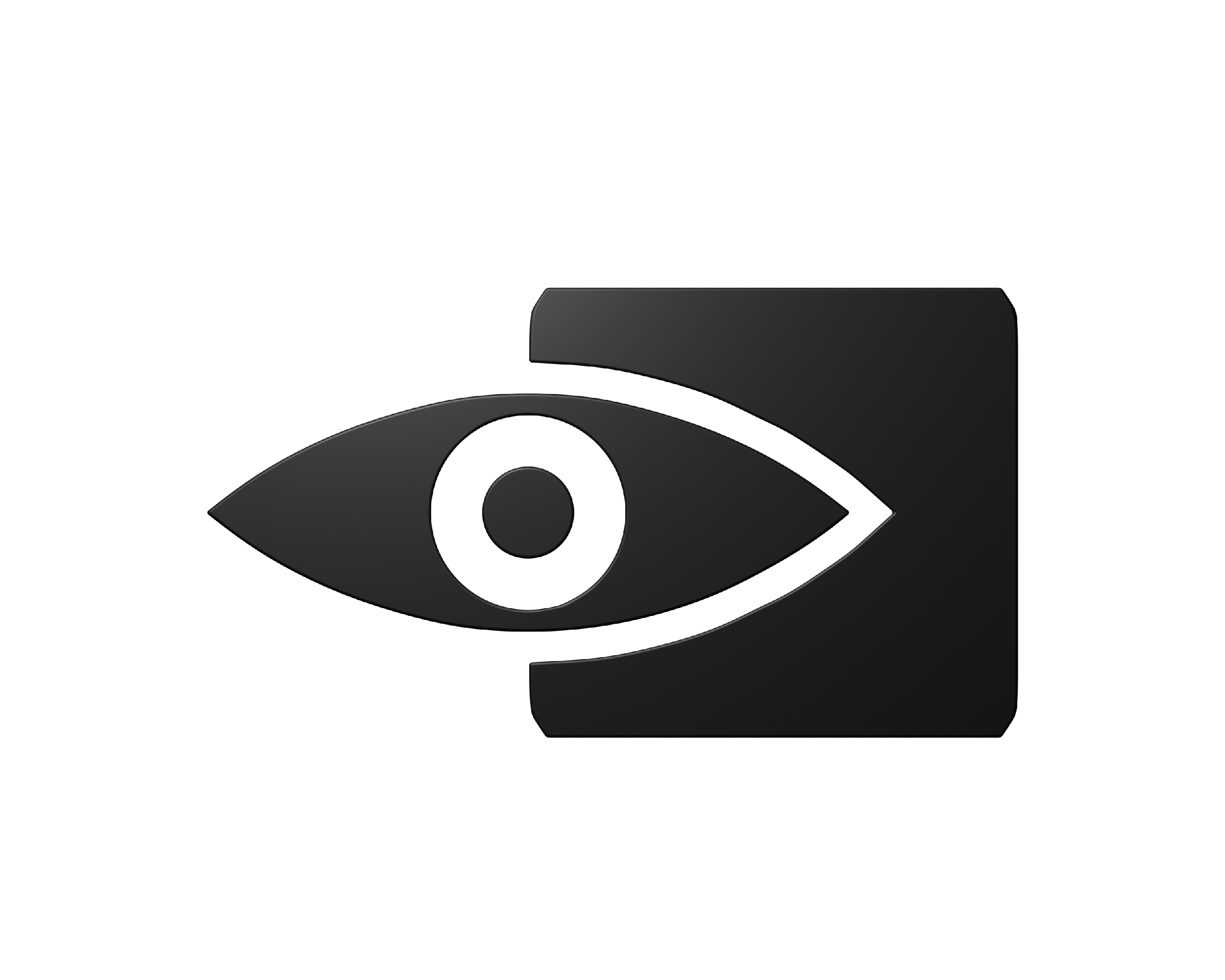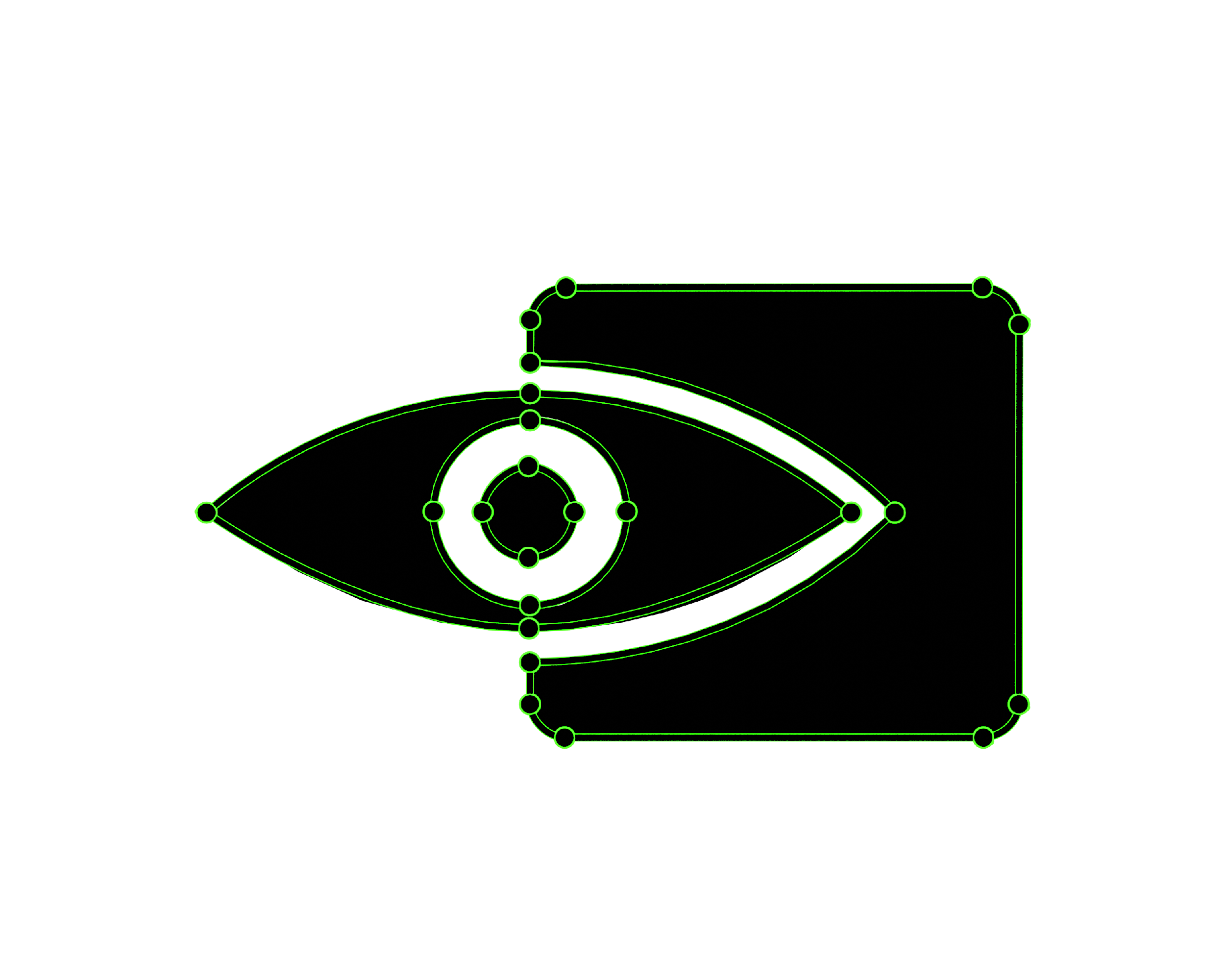## What is a vector?

Computer graphics best friend, the vector has a long storied history stretching back centuries. Starting as the humble tool of mathematicians, used help explain the world around us. Over time its uses have grown to help fill many niches.

The classical definition of a vector is something that contains both a magnitude (or length) and a direction. This definition is a bit confusing on its face so we won't dwell on it too long. What is important to take from this definition is that a vector is a singular object made up of multiple sub parts. A vector composed of two parts is referred to as a 2D vector, a vector with three parts is referred to as 3D vector, four parts 4D, 5 parts 5D, and so on into infinity. For our needs we won't be going beyond 4D vectors.The image on the left side shows the 2D vector points that make up the image on the right. Try clicking the center line to see the difference. The points are connected by bezier curves which give the image its shape and form.

## Applications Of Vectors

Now that we have a basic understand of what makes a vector let’s take a look at some examples of vectors used in computer graphics.

An example of a vector used in computer graphics would be position of an object. Now because we live in the future we can represent position in both the second and third dimension.

A 2D position vector could be represented like this: (X,Y). Where X denotes how far left or right on the screen the object is, while Y denotes how far up or down something is. If you have ever played a 2D Mario game Mario’s position on the screen can be represented by a 2D vector.

A 3D position vector is just a 2D vector with an extra part and can be represented like this: (X,Y,Z). Where just like the 2D vector you have X and Y controlling 2 different directions Z is now a third direction we can go.

While mathematically possible to create and work with a 4D position vector it would confuse our poor little 3D minds so instead we will use color as our example of a 4D vector.

A 4D color vector can represented like this: (R,G,B,A). Unlike the last two vectors where the numbers that made up the parts of the vector could theoretically be any number value the numbers that make up the color vector usually go between 0 and 255. This is due to some computer bit byte nonsense that wont be of importance here, its just the standard. The screen your reading this on is made up of tiny little dots called pixels. Every pixel has with it a 4D color vector telling that pixel what color it should be. The R, G, and B components control how red, green, and blue, a pixel is while the A which stands for alpha controls how transparent or see through the pixel is. for example a (255,0,0,255) is a pure red pixel while the color (255,0,255,255) would be half full red and half full blue so they would mix and form a bright pinkish purple color, and the color (0,255,0,128) would be full green but at half alpha meaning it would blend 50% transparent (there is not actually a pixel behind other pixels some fancy math just blends the two colors together)

This demo shows off how vectors can be used to store information. As you can see this square is moving back and forth on the screen and its position is represented by a 2D vector, where the first component of the vector is the X-coordinate (or where it is left and right on the screen) and the second component is the Y-coordinate (or where it is up and down on the screen). Try hovering over the demo and you will see the square follows your cursor, notice how the X and Y coordinates change when you move the square around.